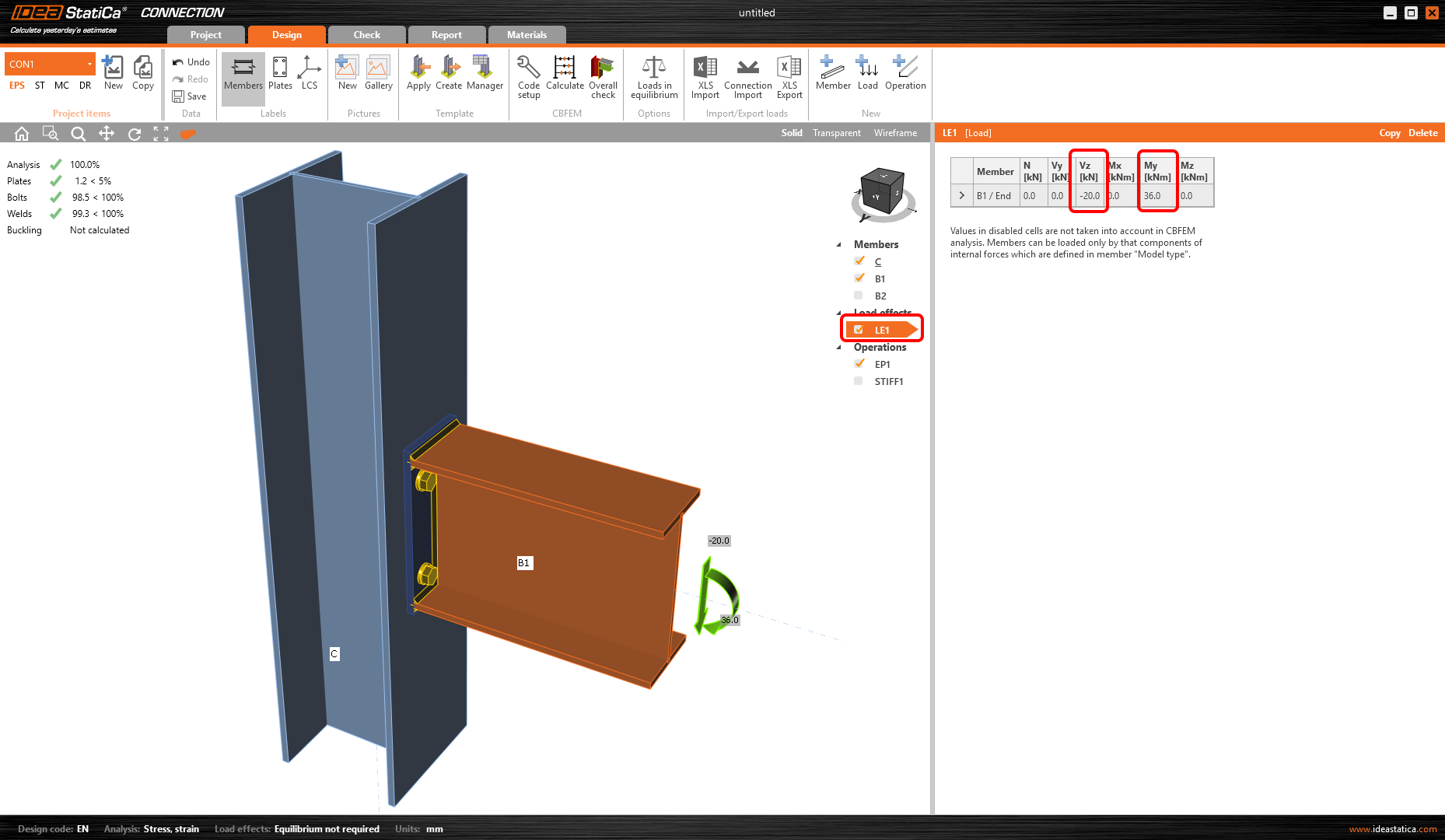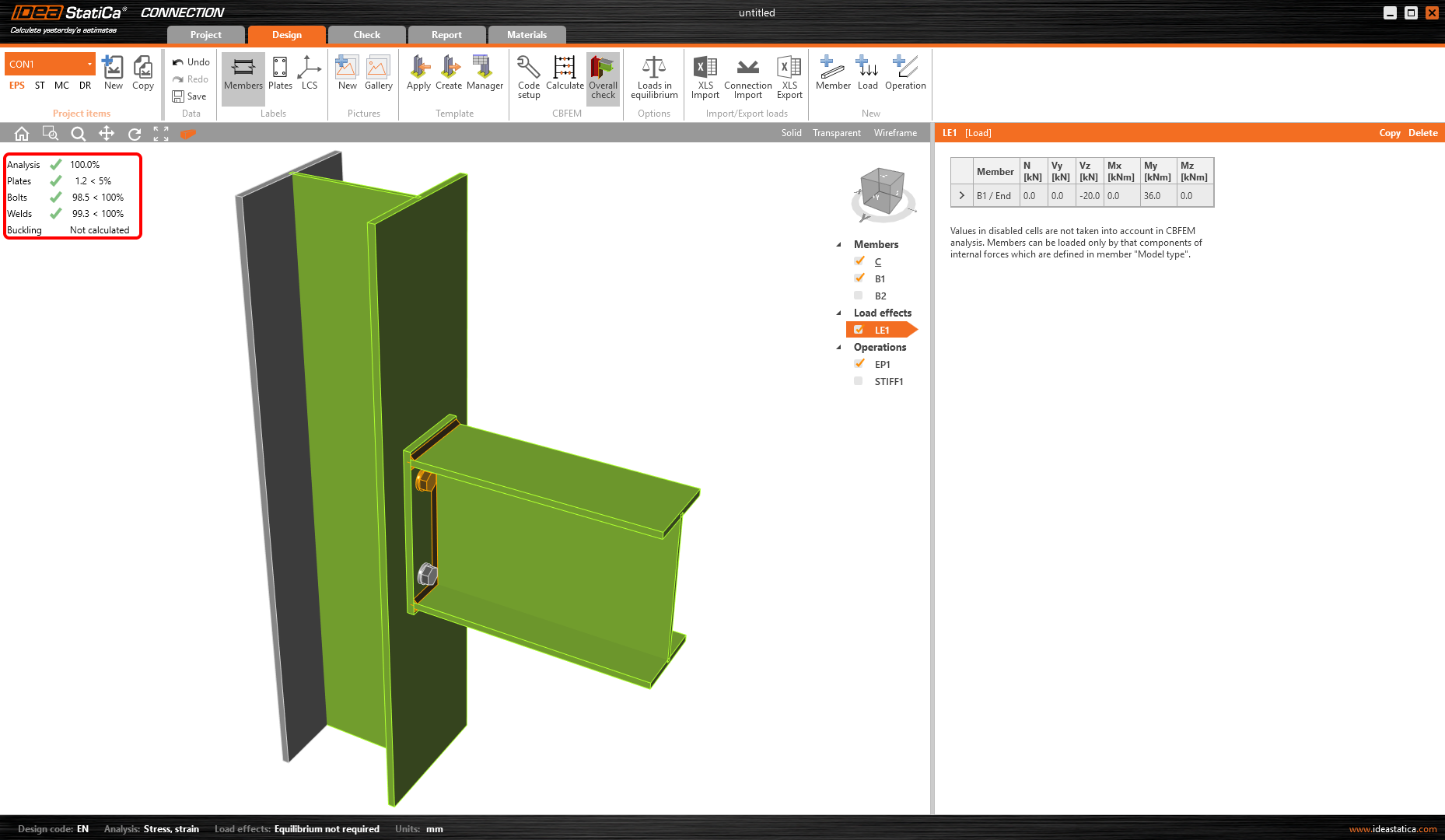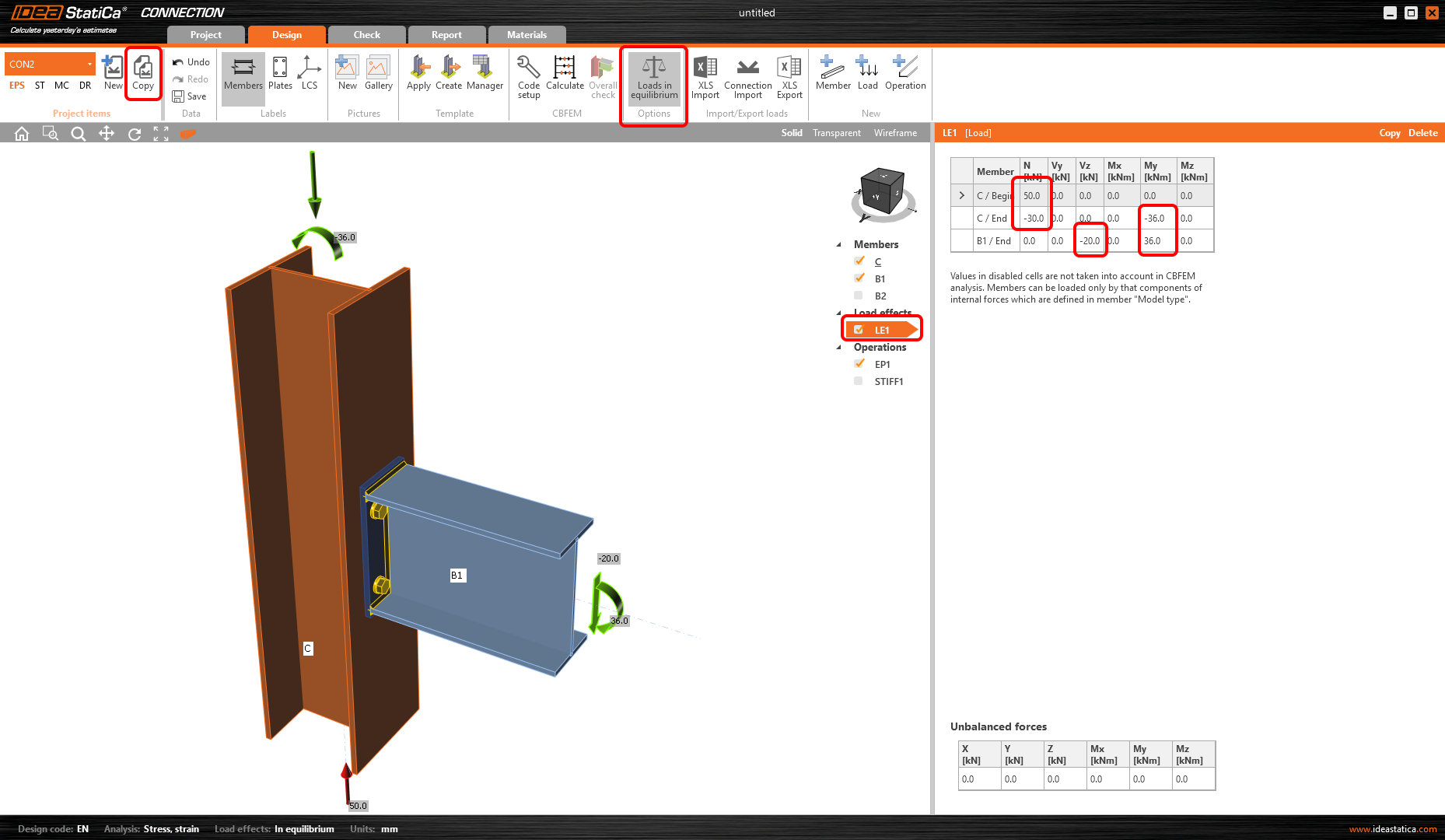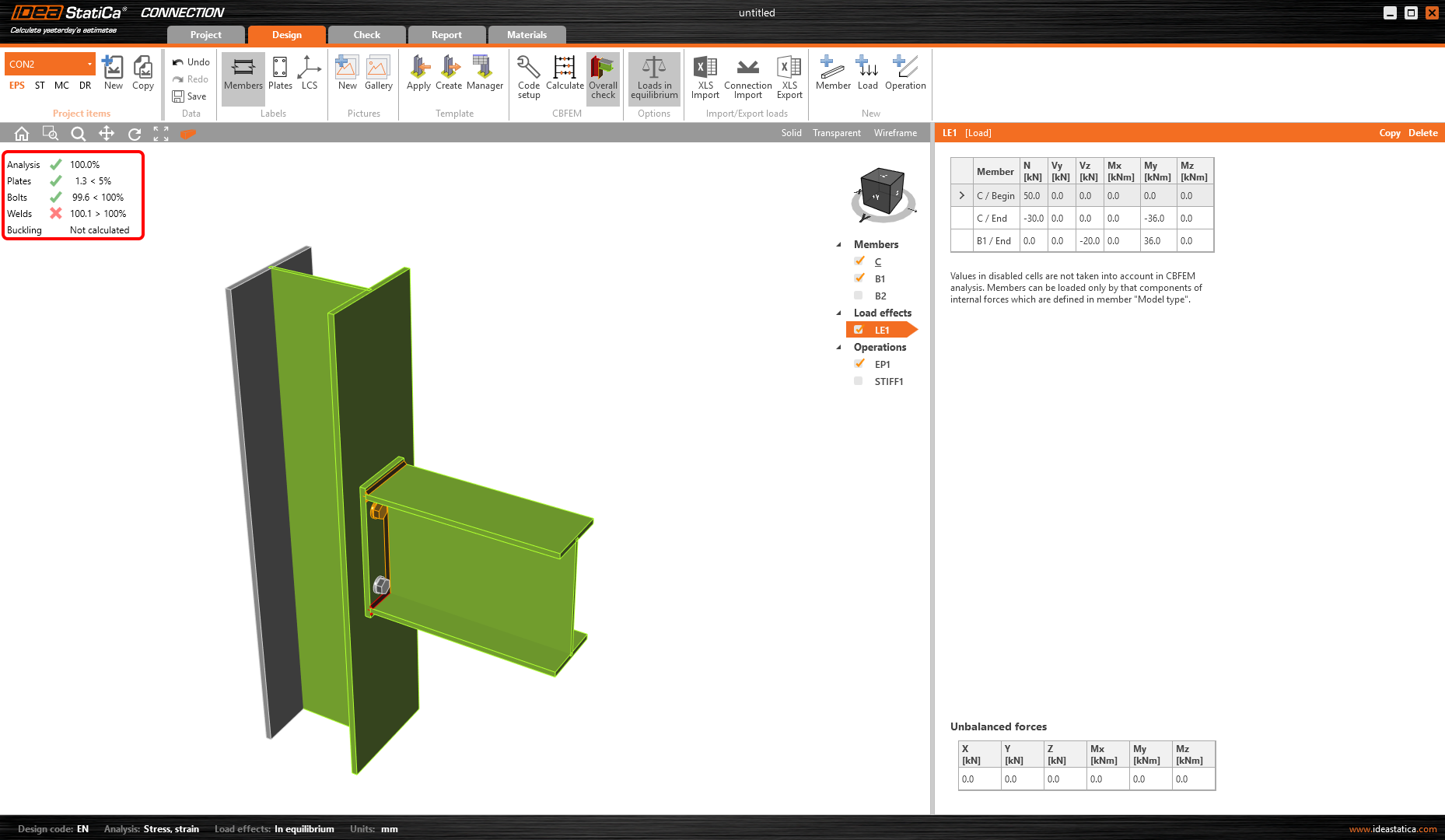### Choose language# Equilibrium and supporting member

$$The internal forces in each node of the frame must be in equilibrium. Especially in the case of a continuous member, we need to carefully input internal forces to all members so the equilibrium is kept in the node..Let’s check an example of a connection of a loaded beam (B1) to a continuous column (C) with Loads in equilibrium option deactivated.After we Calculate the project, the results are provided in the 3D scene. All performed checks are satisfactory.Now, we will Copy the project and activate Loads in equilibrium. The table with Unbalanced forces appears. We can input internal forces on both ends of column C and check the equilibrium of input nodal forces.Again, we Calculate the CON2 project and we see that the welds did not pass the code check. The reason is the correct load action in the column that we considered this time.$$